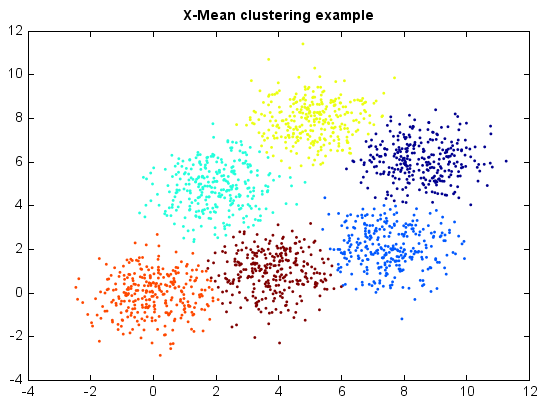# X-Means¶

X-Means clustering algorithm is an extended K-Means which tries to automatically determine the number of clusters based on BIC scores. Starting with only one cluster, the X-Means algorithm goes into action after each run of K-Means, making local decisions about which subset of the current centroids should split themselves in order to better fit the data. The splitting decision is done by computing the Bayesian Information Criterion (BIC).

```from miml import datasets
from miml.cluster import XMeans

fn = os.path.join(datasets.get_data_home(), 'clustering', 'gaussian',
'six.txt')